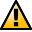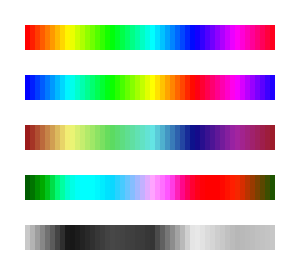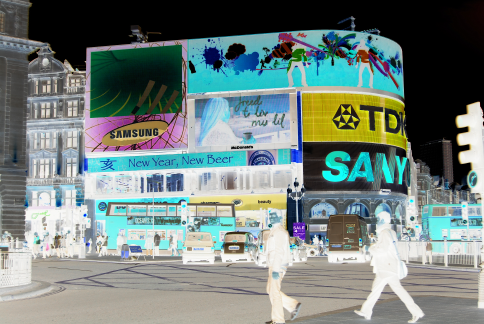WarningThis section not yet updated for Inkscape v0.92.

## Color Filter Primitives

Two Filter primitives allow the manipulation of colors.

WarningThis section not yet updated for Inkscape v0.92.

### Color Matrix

The Color Matrix primitive maps each RGB and Alpha value to a new value. The transformation is described by a 5 × 5 matrix with the bottom row fixed, thus a general transformation is described by a 5 × 4 matrix. The fifth column adds a value that is independent of RGB or Alpha, allowing for nonlinear color correction.Four types of transformations are defined, of which three are special classes of the first.

• Matrix: The full 5 × 4 matrix is defined. This is the most general case.
• Saturate: The saturation is reduced by specifying one number, s. The range of s is 0.0 (completely desaturated) to 1.0 (unchanged). Only the RGB values are changed. The exact formula is:
• R' = (0.213 + 0.787s)R + 0.715 × (1 − s)G + 0.072 × (1 − s)B;
• G' = 0.213 × (1 − s)R + (0.715 + 0.285s)G + 0.072 × (1 − s)B;
• B' = 0.213 × (1 − s)R + 0.715 × (1 − s)G + (0.072 + 0.928s)B.
• Hue Rotate: The hue is shifted by specifying one number. Like the Saturate case, only RGB values are changed. The exact formula is quite complicated. It is not just a red to yellow to green and so on rotation.
• Luminance to Alpha: The luminance is converted to Alpha via a fixed formula: Alpha = 0.2125 × R + 0.7154 × G + 0.0721 × B (from ITU-R Recommendation BT709, the HDTV color standard).Examples of using the Color Matrix primitive. From top to bottom: Source object. Matrix mode set to swap red and blue. Saturate mode with input of 0.5. Hue Rotate mode with input of 90°. Luminance to Alpha mode.

A negative can be made by setting the RGB diagonal matrix elements (a00, a11, a22) to −1.00 and the top three elements of the fifth column (a04, a14, a24) to 1.00.Creation of a negative using the Matrix mode.
WarningThis section not yet updated for Inkscape v0.92.

### Component Transfer

Partially implemented, No user interface.

The Component Transfer primitive changes the RGB and Alpha of an object by applying independent functions to each of the RGB and Alpha input values. The following modes for defining the functions are available: Identity, Table, Discrete, Linear, and Gamma.